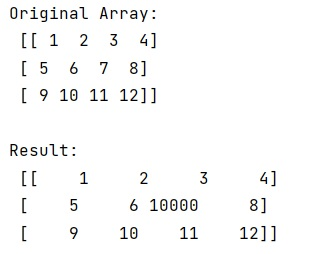# How to change a single value in a NumPy array?

Learn, how to change a single value in a NumPy array in Python?
Submitted by Pranit Sharma, on February 20, 2023

NumPy is an abbreviated form of Numerical Python. It is used for different types of scientific operations in python. Numpy is a vast library in python which is used for almost every kind of scientific or mathematical operation. It is itself an array which is a collection of various methods and functions for processing the arrays.

## Changing a single value in a NumPy array

Suppose that we are given a numpy ndarray and we need to change a single element of this array.

For example, if we are given arr = [[1,2],[1,2]] and we need to change 2 in first row with 3.

For this purpose, we have a simple approach. We just need to index the element and assign a new value. While indexing, we must make sure that the coordinates are correct and also not out of bounds.

Also, in numpy, we can index an element using [x,y] or [x],[y] and it does not throw an error on this.

Let us understand with the help of an example,

## Python code to change a single value in a NumPy array

```# Import numpy
import numpy as np

# Creating a numpy array
arr = np.array([[1,2,3,4],[5,6,7,8],[9,10,11,12]])

# Display original array
print("Original Array:\n",arr,"\n")

# Replacing 7 in 2nd row with 10000
arr = 10000

# Display result
print("Result:\n",arr)
```

Output: